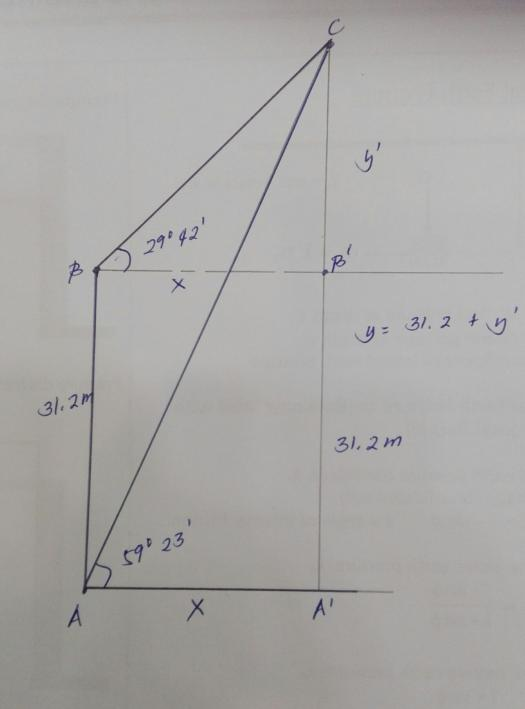# Angle of Elevation

6 posts / 0 new
esmilitarAngle of Elevation

The angle of elevation of a point C from a point B is 29042'; the angle of elevation of C from another point A 31.2 m directly below B is 59023'. How high is C from the horizontal line through A?

Jhun Vert$\alpha = 90^\circ - 59^\circ 23' = 30^\circ 37'$

$\beta = 90^\circ + 29^\circ 42' = 119^\circ 42'$

$\theta = 180^\circ - \alpha - \beta = 29^\circ 41'$$\dfrac{x}{\sin \beta} = \dfrac{31.2}{\sin \theta}$

$x = 54.73 ~ \text{m}$

$y = x \sin 59^\circ 23'$

$y = 47.098 ~ \text{m}$       answer

esmilitarThanks a lot sir its a great help.

esmilitarThanks a lot sir its a great help. I had another solution using tangent got the same answer..

esmilitarAB=A'B'=31.2m
AA'=BB'=X
A'C=y=31.2 + y'
y=31.2 + y'

tan 29042'=y'/x

x = y'/ tan 29042'
tan 59023'=y/x
tan 59023'=(31.2+y')/(y'/(tan 29042')
(tan 59023')(y')/(tan29042')=31.2 +y'
2.9625y'-y'=31.2
y'=31.2/1.9625
y'=15.898m
y=31.2 + 15.898
y=47.098m Ans

Jhun VertYes. also an straightforward solution.

• Mathematics inside the configured delimiters is rendered by MathJax. The default math delimiters are $$...$$ and $...$ for displayed mathematics, and $...$ and $...$ for in-line mathematics.